# Place Value - Definition with Examples

The Complete K-5 Math Learning Program Built for Your Child

• 30 Million Kids

Loved by kids and parent worldwide

• 50,000 Schools

Trusted by teachers across schools

• Comprehensive Curriculum

Aligned to Common Core

## Place Value Games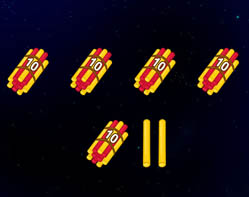Numbers up to 100

Develop the understanding of place value of numbers from 51 to 99 by learning that a 2 digit number is a number made up of tens and ones.

Covers Common Core Curriculum 1.NBT.2

## What is Place Value?

In math, every digit in a number has a place value.
Place value can be defined as the value represented by a digit in a number on the basis of its position in the number.

Here’s an example showing the relationship between the place or position and the place value of the digits in a number.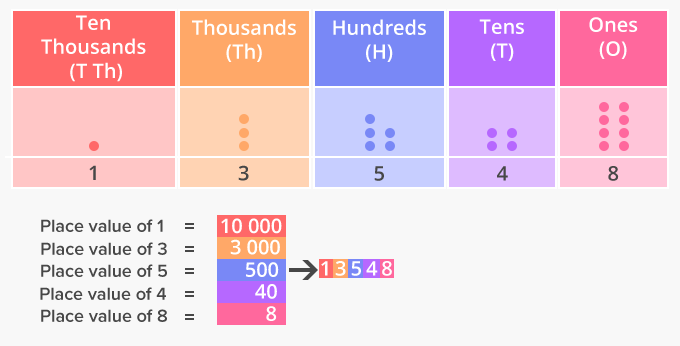In 13548, 1 is in ten thousands place and its place value is 10,000,
3 is in thousands place and its place value is 3,000,
5 is in hundreds place and its place value is 500,
4 is in tens place and its place value is 40,
8 is in ones place and its place value is 8.

Understanding the place value of digits in numbers helps in writing numbers in their expanded form. For instance, the expanded form of the number above, 13548 is 10,000 + 3,000 + 500 + 40 + 8.

A place value chart can help us in finding and comparing the place value of the digits in numbers through millions. The place value of a digit increases by ten times as we move left on the place value chart and decreases by ten times as we move right.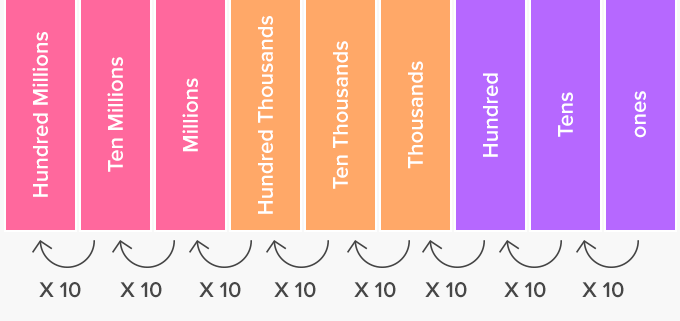Here’s an example of how drawing the place value chart can help in finding the place value of a number in millions.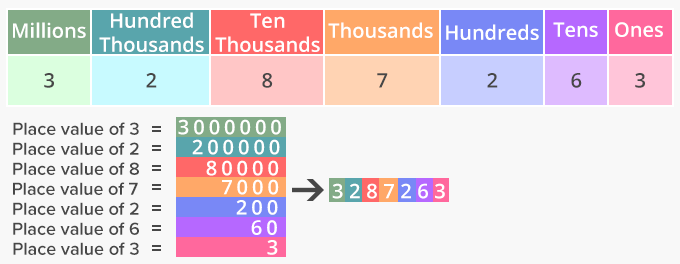In 3287263, 3 is in millions place and its place value is 3000000,
2 is hundred thousands place and its place value is 200000,
8 is in ten thousands place and its place value is 80000,
7 is in thousands place and its place value is 7000,
2 is in hundreds place and its place value is 200,
6 is in ten place and its place value is 60,
3 is in ones place and its place value is 3.

The place value of digits in numbers can also be represented using base-ten blocks and can help us write numbers in their expanded form.

Here’s how the number 13548 can be represented using base-ten blocks.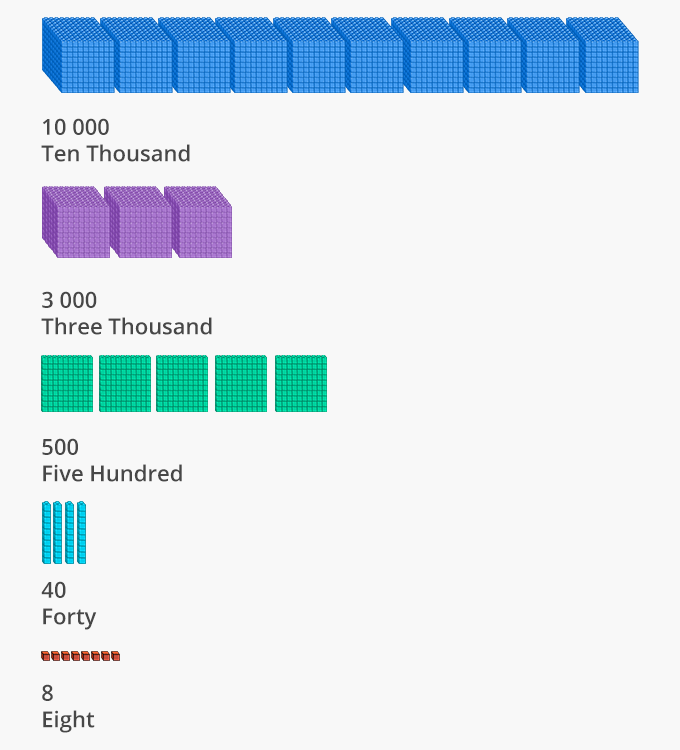Decimal Place Value
Decimal numbers are fractions or mixed numbers with denominators of powers of ten. In a decimal number, the digits to the left of the decimal point represent a whole number. The digits to the right of the decimal represent the parts. The place value of the digits becomes 10 times smaller.

The first digit on the right of the decimal point means tenths i.e..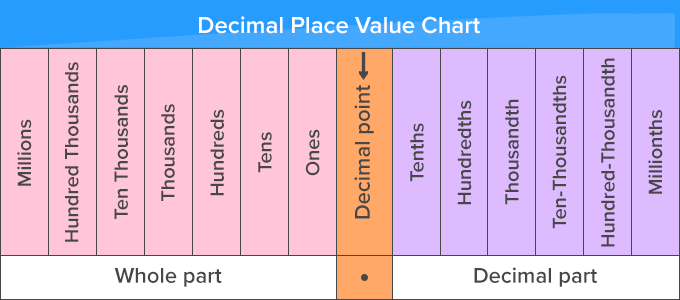In 27.356, 27 is the whole number part,
2 is in tens place and its place value is 20,
7 is in ones place, and its place value is 7.

There are three digits to the right of the decimal point,

3 is in the tenths place, and its place value is 0.3 or,

5 is in the hundredths place, and its place value is 0.05 or,

6 is in the thousandths place, and its place value is 0.006 or.

 Fun Facts Place value and face value are not the same. The face value of a number is the value of the digit or numeral itself. For instance, the face value of 2 in 12783 is 2.Let's sing!

There’s a place value that the digits hold,
Basis its place in the number, I am told.
The ones are the digits we see on the right,
Next we move left to the tens in sight.
Then, are the hundreds standing tall and bright.Let's do it!

Instead of teaching place value through lessons and learning videos and giving worksheets to your 2nd grade children, Take a walk with your child around the neighbourhood. Look for one-, two-, three-, and four digit numbers. Have the child read them aloud. Discuss each number and ask how many ones, tens, hundreds, or thousands are in the number. You can further ask them to compare the numbers and find the largest and smallest number.Related math vocabulary

Won Numerous Awards & Honors